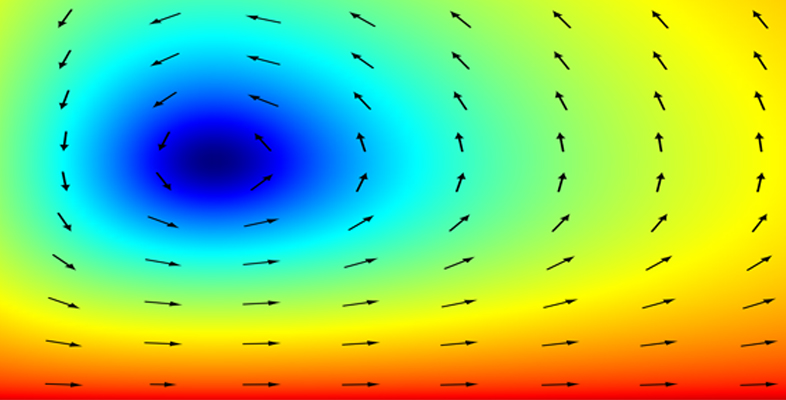Dynamics

Start this free course now. Just create an account and sign in. Enrol on the course to track your learning.

Free course

# Dynamics

## Introduction

Please note: a Statement of Participation is not issued for this course.

This free course, Dynamics, is an adapted extract from the Open University course MST210 Mathematical methods, models and modelling [Tip: hold Ctrl and click a link to open it in a new tab. (Hide tip)] , a core second level applied mathematics course for several mathematics and mathematics-related degrees. The course is designed to teach ‘pencil and paper’ techniques that are at the core of applied mathematics. Students are expected to be familiar with standard mathematical notation and with elementary functions such as sines, cosines, logarithms and exponentials. Familiarity with algebra, calculus and complex numbers is also assumed.

Dynamics consists of material from MST210 Unit 3, Dynamics and has five sections in total. You should set aside between three to four hours to study each of the sections; the whole extract should take about 16 hours to study. The extract is a small part (around 5%) of a large course that is studied over eight months, and so can give only an approximate indication of the level and content of the full course.

This extract is one of the core mechanics units from MST210 in which we look at moving objects, i.e. dynamics. It is expected that you have had an introduction to Newtonian mechanics prior to study of this extract. The starting point is Newton’s laws of motion. To apply these laws, we need to be able to translate them into useful mathematical equations. The extract takes an approach of illustrating techniques alongside their major applications; this is typical of MST210. It is relatively self-contained and should be reasonably easy to understand for someone who has not studied any of the previous texts in the course. However, a few techniques and definitions taught in earlier units in MST210 are present in the extract without explanation.

Mathematical/statistical content at the Open University is usually provided to students in printed books, with PDFs of the same online. This format ensures that mathematical notation is presented accurately and clearly. The PDF of this extract thus shows the content exactly as it would be seen by an Open University student. However, the extract isn't entirely representative of the module materials, because there are no explicit references to use of the MST210 software or to video material (although please note that the PDF may contain references to other parts of MST210). In this extract, some illustrations have also been removed due to copyright restrictions.

Regrettably, mathematical and statistical content in PDF form is not accessible using a screenreader, and you may need additional help to read these documents.

Section 1 introduces concepts like position, velocity and acceleration, which describe the way an object moves.

Section 2 discusses Newton’s laws of motion, which predict the motion of an object when the forces acting on it are known.

Section 3 shows how Newton’s second law of motion can be used to predict the motion of objects.

Section 4 concerns modelling some of the forces that occur in nature, which enables more realistic situations to be analysed.

Section 5 looks at motion in more than one dimension.

This OpenLearn course is an adapted extract from the Open University course MST210 Mathematical methods, models and modelling.

MST210_1